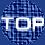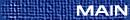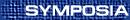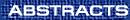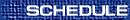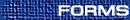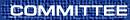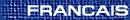Matrix Analysis / Analyse matricielle
(Judith McDonald, Organizer)

ROD EDWARDS, University of Victoria, Victoria, British Columbia  V8W 3P4
Common invariant proper cones and ray dynamics

Certain problems in dynamics of networks give rise to dynamical systems in which matrix operators act on the space of rays in Rn. One important problem is to find conditions on a pair (or set) of real matrices such that rays become localized under the action of `words' composed of multiple products of these matrices, and to find regions of Rn where they are confined. If eigenvalues of the matrices are real, for example, one may look for conditions giving a common invariant proper cone, K, for the two matrices. In general, this problem appears to be hard, but we present some partial results including a sufficient condition in the case of R3. Negative dominant eigenvalues may still allow invariant sets of the form KÈ(-K), where K is a proper cone, and dominant complex eigenvalues may allow other forms of invariant set.

SHAUN FALLAT, Department of Mathematics and Statistics, University of Regina, Regina, Saskatchewan  S4S 0A2
Eigenvalues of products of matrices and submatrices in certain positivity classes

If A and B are n-by-n, positive semidefinite Hermitian matrices, then

 lmin(AB) £ lmin(A[a]B[a]),
for any f ¹ a Í {1,2,¼,n}. A certain converse is given, as well as analogs for the product of several M-matrices and totally nonnegative matrices. However, analogs for lmax (other than the case of totally nonnegative matrices) and for singular values of general matrices, etc., fail.

CHUN-HUA GUO, University of Regina, Regina, Saskatchewan
Iterative methods for a nonlinear matrix equation

We are concerned with the numerical computation of the maximal Hermitian positive definite solution of the matrix equation X+A*X-1A=Q, where Q is Hermitian positive definite. Three iterative methods are discussed: a simple fixed point iteration, the Newton method, and a method based on cyclic reduction.

ALLEN HERMAN, University of Regina, Regina, Saskatchewan
A maximal eigenvalue conjecture equivalent to permanental dominance

Using concepts from the character theory of finite groups, a conjecture about maximal eigenvalues will be presented that is equivalent to Lieb's permanental dominance conjecture.

HADI KHARAGHANI, University of Lethbridge, Lethbridge, Alberta
w-shift matrices and applications

Let Cn=\prec w\succ be a cyclic group of order n. The matrix UW, where U is the circulant matrix of order n with first row (0,1,0,¼,0) and W = diag(w,1,¼,1), is called an w-shift matrix. Let q be a prime power and n a positive integer with [(q-1)/(n)] being an even (respectively odd) integer. Using a cyclic group of w-shift matrices, we show that there is a symmetric (respectively skew) BGW(1+q+¼+q2m+1,q2m+1,q2m+1-q2m) with zero diagonal over the cyclic group Cn of order n, for each nonnegative integer m. Applications include a large class of new (respectively directed) Strongly Regular Graphs.

STEVE KIRKLAND, University of Regina, Regina, Saskatchewan  S4S 0A2
A bound on algebraic connectivity for graphs with cutpoints

For a graph G, its Laplacian matrix can be written as L=D-A, where A is the (0,1) adjacency matrix of G, and D is the diagonal matrix of vertex degrees. The algebraic connectivity of G is the second smallest eigenvalue of L, and as the name suggests, it seems to provide an algebraic measure of the connectedness of G. A vertex of G is a cutpoint if its deletion disconnects the graph. In this talk, we consider graphs on n vertices with k cutpoints, provide a tight upper bound on the algebraic connectivity in terms of n and k, and characterize the graphs yielding equality in the bound.

CHI-KWONG LI, William and Mary, International Linear Algebra Society Speaker, Williamsburg, Virginia  23187, USA
Linear Preserver Problems: A gentle introduction and some recent results

Linear preserver problems concern the characterizations of linear transformations on matrix or operator spaces that have some special properties. In this talk, a gentle introduction of the subject including history, motivations of study, and some current research will be presented. Recent results obtained by the speaker and his collaborators will also be described.

GORDON MACDONALD, University of Prince Edward Island, Charlottetown, Prince Edward Island
N-transitivity and the complementation property

The structure of (minimal) n-transitive rank-n semigroups of k×k matrices of is investigated by considering an equivalent problem regarding certain families of (k-n)-dimensional subspaces of the underlying vector space.

JOHN MAROULAS, National Technical University of Athens
On compressions of normal matrices

Let A be a normal matrix and consider the polygon NR[A]={x*Ax:||x||=1 }. If u*Au Î NR[A], a projector matrix Pu is defined such that NR[P*uAPu] is supported by all or some edges of the polygon. The inverse problem for a matrix G, and the dilation of NR[G] to a circumscribed hexagon are also investigated.

CARL MEYER, Department of Mathematics, North Carolina State University, SIAM Speaker
Replacing the SVD in LSI and information retrieval applications

Latent semantic indexing (LSI) is a vector space technique that utilizes the SVD to process queries in an information retrieval system such as an internet search engine. Some of the bottlenecks associated with the SVD approach include storage considerations, difficulties in updating and downdating the underlying data base, and speed vs. reliability issues. After reviewing some of these basic problems, some different vector space approaches to LSI that do not involve an SVD decomposition will be presented and discussed.

DALE OLESKY, Department of Computer Science, University of Victoria, Victoria, British Columbia
Successively-ordered elementary bidiagonal factorization

Let D be a diagonal matrix and Eij denote the n-by-n matrix with a 1 in entry (i,j) and 0 in every other entry. An n-by-n matrix A has a successively-ordered elementary bidiagonal (SEB) factorization if it can be factored as

 A = æè n-1Õ k=1 k+1Õ j=n Lj(sjk) öø D æè 1Õ k=n-1 nÕ j=k+1 Uj(tkj) öø ,
in which Lj(sjk)=I+sjkEj,j-1 and Uj (tkj) = I+tkjEj-1,j for some scalars sjk, tkj. Note that some of the parameters sjk,tkj may be zero, and the order of the bidiagonal factors is fixed. If this factorization corresponds to reduction of A to D via successive row/column operations in the specified order, it is called an elimination SEB factorization. New rank conditions are formulated that are proved to be necessary and sufficient for matrix A to have such a factorization. These conditions are related to known but more restrictive properties that ensure a bidiagonal factorization as above, but with all parameters sjk, tkj nonzero.

P.N. SHIVAKUMAR, University of Manitoba, Winnipeg, Manitoba  R3T 3N2
Some nonsingular matrices and their applications

Nonsingular matrices with various structures involving certain sign patterns, diagonal dominance, tridiagonal, etc., are presented. Applications include, extensions to the infinite case, Digital Circuit Dynamics, conformal mapping of doubly connected regions and double points of a Mathieu equation.

MICHAEL TSATSOMEROS, University of Regina, Department of Mathematics and Statistics, Regina, Saskatchewan  S4S 0A2
Perron-Frobenius type results on the numerical range

We present results connecting the shape of the numerical range to intrinsic properties of a nonnegative matrix A. These results are to a large extent analogous to the Perron-Frobenius theory, especially as it pertains to irreducibility and cyclicity in the combinatorial sense. Special attention is given to circular and elliptic numerical ranges.

PETER ZIZLER, Mount Royal College, Calgary, Alberta
Norms of sampling operators

Let h(z) be an essentially bounded complex valued function on the unit circle T={z| |z|=1}. Consider the corresponding Laurent operator Lh=(hn-m)n,m Î Z, where hn is the n-th Fourier coefficient of h(z), hn=òT h(z)z-n dz.

Let us consider an operator Sh(p,q), which we shall call a sampling operator, defined as Sh(p,q)=(hpn-qm)n,m Î Z, where p,q Î N={1,2,... }. These operators are obtained from Lh be ``keeping'' every q-th column and every p-th row in the bi-infinite matrix Lh. In our paper, we find an upper and lower bound for the norm of the operator Sh(p,q).